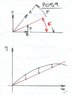# Cannon and Hill Problem

• skeer

#### skeer

My problem goes :

A projectile is fired with initial speed v_0 at an angle of \alpha up a hill slope \beta (\alpha > \beta)
a) How far up the hill will the projectile land.

Attempt to solution:

I understood that this is a problem where I need to find the intersection of the parabola and the line.

the function of the line is y= x tan (\beta)

The parabola is y = [gx^2+2x(v_0)^2cos (\alpha)sin(\alpha) ]/ [ 2(v_0)^2 (\alpha)]

After making them equal to each other I get

x= [2 (v_0)^2 cos (\alpha) sin (\alpha- \Beta) ] / gcos (\beta)

This is almost the answer... but somewhere I made a mistake or I am missing an step. Is my approach correct?

P.S. The answer is d= [2 (v_0)^2 cos (\alpha) sin (\alpha- \Beta) ] / g cos^2 (\beta)

Last edited:

The parabola is y = [gx^2+2x(v_0)^2cos (\alpha)sin(\alpha) ]/ [ 2(v_0)^2 (\alpha)]
##y = [gx^2+2x{v_0}^2\cos (\alpha)\sin(\alpha) ]/ [ 2{v_0}^2 (\alpha)]##
You've obviously left out a trig function in the denominator.
Restore that, then check it by e.g. evaluating y'(0).

I am new on the forum and I wasn't clear how to write formulas with LateX. I will from now on.

Also, I ask some of my friends in class how they solved the problem. My issue was that I was taking the displacement along the x; when actually I need to find the displacement along the hill ( or in other words the hypotenuse of the angle $\beta$)

Imagine a point on the hill directly below the projectile, this point has a constant vertical velocity during the flight ( you can figure it from the projectile behaviour )
Then you can ignore horizontal activity and treat the problem as following :
The projectile and the point are launched vertically at the same time, the projectile having the greater velocity ( which is the vertical component of the launch velocity )

Have a look at this sketch :
A = launch velocity in m/s
B = ( constant ) horizontal vector in m/s = cosine F * A
C = vertical vector in m/s = sine F * A
D = ( constant ) vertical velocity of point on incline directly below the projectile = tan E * B
E = incline angle in degrees
F = launch angle in degrees
t = elapsed time in seconds
For impact point :
( D * t ) = ( C * t ) + ( ½ * - g * t ² )
Then :
( D * t ) - ( C * t ) = ½ * - g * t ²
D – C = ( ½ * - g * t ² ) / t
And :
( D – C ) / ( ½ * - g ) = t

With t you can locate the impact point x and y coordinates

#### Attachments

•p059.jpg
17.5 KB · Views: 380
Thank dean barry for replying! That is what I was doing at the begging since I understood from the problem that the wanted the height (the value of y). But what the problem asked was to find the distance from the bottom of the hill to the hitting point along the hill. They are asking for the distance "d" in my drawing.
I solve the problem already :) . If you are curious and would like to see my work, let me know and I can upload a picture of it.